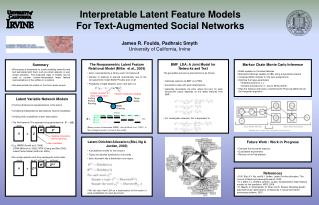Download PresentationSummary

# Summary

Download Presentation## Summary

- - - - - - - - - - - - - - - - - - - - - - - - - - - E N D - - - - - - - - - - - - - - - - - - - - - - - - - - -
##### Presentation Transcript

1. Interpretable Latent Feature Models For Text-Augmented Social Networks James R. Foulds, Padhraic SmythUniversity of California, Irvine • The Nonparametric Latent Feature Relational Model (Miller et al., 2009) • Actor i represented by a binary vector of features Zi • Number of features K learned automatically due to the non-parametric Indian Buffet Process prior on Z • Probability of edge between actor i and actor j is • Binary matrix factorization (BMF) , due to Meeds et al. (2007), is the rectangular matrix version of this model. • BMF_LDA: A Joint Model for Networks and Text • The generative process is assumed to be as follows: • Generate network via BMF (or LFRM) • Associate a topic with each latent feature • Generate documents via LDA, where the prior for each document’s topics depends on the latent features from BMF: • For rectangular networks, this is equivalent to: • Markov Chain Monte Carlo Inference • Gibbs updates on the latent features • Metropolis-Hastings updates for Ws, using a Gaussian proposal • Collapsed Gibbs sampler for the topic assignments • Optimize the hyper-parameters • Gradient ascent for λ, γ • Iterative procedure for α+, due to Minka (2000). • Align the features and topics, maximizing the Polya log-likelihood via the Hungarian algorithm. • Summary • We propose a framework for jointly modeling networks and text associated with them, such as email networks or user review websites. The proposed class of models can be used to recover human-interpretable latent feature representations of the entities in a network. • We demonstrate the model on the Enron email corpus. • Latent Variable Network Models • Find low-dimensional representations of the actors • Conditional independence assumptions improve tractability • Unifying view: probabilistic matrix factorization • The NxN network Y is assumed to be generated via • E.g. MMSB (Airoldi et al. 2008), • LFRM (Miller et al. 2009), RTM (Chang and Blei 2009), • Latent Factor Model (Hoff et al. 2002),… • Two mode networks and other rectangular matrix data: NxM NxN NxK(1) NxK K(1)xK(2) KxK K(2)xM KxN W W Z(2)T ZT Λ Λ = = Z(1) Z Feature Z = Actor Y ∼ f(Λ), Variable interaction terms (optional) • Latent Dirichlet Allocation (Blei, Ng & Jordan, 2003) • A probabilistic model for text corpora • Topicsare discrete distributions over words • Each document has a distribution over topics • We can also view LDA as a factorization of the matrix of word probabilities for each document. • Future Work / Work in Progress • Evaluate the recovered features • Quantitative experiments • Results on the Yelp dataset Latent variables • References • D.M. Blei, A.Y. Ng, and M.I. Jordan. Latent Dirichlet allocation. The Journal of Machine Learning Research, 2003. • K.T. Miller, T.L. Griffiths, and M.I. Jordan. Nonparametric latent feature models for link prediction. NIPS, 2009. • E. Meeds, Z. Ghahramani, R. Neal, and S. Roweis. Modeling dyadic data with binary latent factors. In Advances in neural information processing systems, 2007. Cycling Fishing Running Tango Salsa B A C Waltz Running Logistic function (or other link function) Feature interaction weights